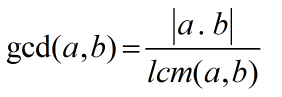Request a Tool

GCF Calculator

GCF Calculator is use to calculate greatest common factor of two input values.

GCF
0

Formula• gcd = greatest common divisor
• lcm = least common multiple
• a = First Value
• b = Second Value

Defination / Uses

A collection of numbers' greatest common factor (GCF) is the largest factor that all of the numbers share. Twelve, twenty, and twenty-four, for example, all have two common factors: two and four. The GCF of 12, 20, and 24 equals 4 since the largest is 4. The GCF method is frequently used to detect common denominators.

The largest positive integer that divides two non-zero integers without leaving a residual is the greatest common factor (GCF). The largest common denominator is also known as the highest common factor (HCF) or greatest common divisor (GCD). Factorization groups the characteristics into a single factor. All of the factors are detachable from a single numeric number. The factorization also leads to the Greatest Common Factor categorization for common factors. Use a free factor calculator to determine only the factor.